# Summary Method - Multiply/ProductHi all,

I currently have one line item with 2 dimensions, and the numbers are percentages. I essentially want to **** out one of the dimensions in a new line item but to have all the percentages multiply by each other for the dimension that was taken out.

In simple terms, I'm looking for a 'Summary Method' that multiplies rather than a sum / formula / average etc.

Does anyone know how this can be done? I've attached an Excel which shows what I want to get to in the line item. Apologies if it's something completely obvious. 🙂

Many thanks

Tagged:

•Are the percentages  in your current View/Table calculated or input? And How are you calculating the percentages in your Result Table?

•Hi Misbah,

The percentages in my current view are all calculated with a formula from other different inputs. The result table formula is the issue I'm having as I'm not sure how to multiply the percentages with the other percentages from the other items in the dimension.

If there was a 'Multiply' option as a Summary Method for example, that would solve my issue.

Thanks

•Hello @sd12901 @Misbah,

I have a solution that is a bit of a workaround. This workaround entails 3 steps.

1. I created the module which has percentagtes dimensionalized by Dimension 1 x Dimension 2.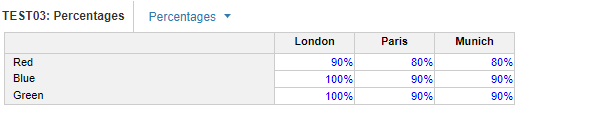2. I created a "Constants" module, that has no dimensions, only line items. The line items are list formatted as Dimension 2.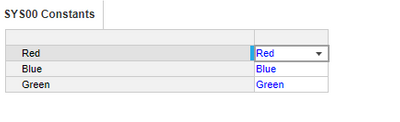3. I created a module dimensionalized by Dimension 1, line item called "Average Percentages".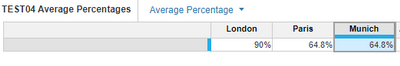Formula: 'TEST03: Percentages'.Percentages[LOOKUP: 'SYS00 Constants'.Green] * 'TEST03: Percentages'.Percentages[LOOKUP: 'SYS00 Constants'.Blue] * 'TEST03: Percentages'.Percentages[LOOKUP: 'SYS00 Constants'.Red]

Considerations:

-This method requires the maintanence of a Constants module. My initial idea was to use SELECT statements but this goes against the Planual principle avoid hardcoding formulas. So instead used a Constants module and performed the multiplication manually.

Let me know if this helps!

I am going to keep playing around with the summary methods and different logic/line items to see if there is a way to do this without using a Constants module.

•I do have another method for you. In addition to the above method you can try this:

1. 1. I created the module which has percentages dimensionalized by Dimension 1 x Dimension 2. Summary Method: Average.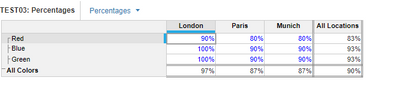2. I have a 2nd line item in the same module, called "Average". Summary Method: Formula.

Formula: Percentages * Percentages * Percentages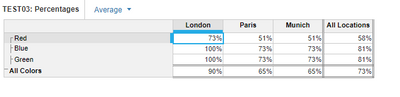3. I have another module dimensionalized by Dimension 1. Line Item: Avg. Percentage.

Formula: 'TEST03: Percentages'.Average.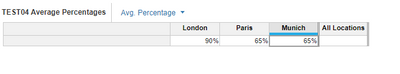Considerations:

This method does not require the manual maintanence of a Constants Module. The multiplication of 3 percentages in Step 2, is something that would have to be maintained as when you have to add more colors I believe.

Hopefully this helps!

•Thanks a lot for the detailed explanation and for taking the time to investigate! I do have a lot more items in each dimension than my initial example so I may look to explore a way to not have a long formula.

If I can't find anything, I may use this example as the list items are unlikely to change regularly and it could just be a one-off long formula to begin with.

Much appreciated.

•Hi @sd12901@Misbah

No problem! I made 1 last attempt at the formula, to see if there is a way to automate this without any maintanence. I think I found something you will like!

1. I made a module dimensionalized by Dimension 1 x Dimension 2. Line Item: Percentages. Summary Method: Average.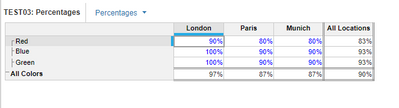2. I made a System module dimensionalized by Dimension 2. Line Item: Count. Summary Method: Sum.

Formula: "1".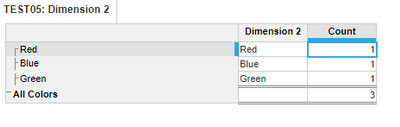3. I created a module dimensionalized by Dimension 1. Line Items: Avg Percentage. Summary: none.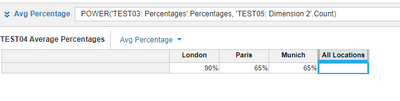Formula: POWER('TEST03: Percentages'.Percentages, 'TEST05: Dimension 2'.Count)

I gave some thought to some of my previous responses and noticed that I am essentially multiplying "average percentage" by itself "X" number of times. Where X = the count of Colors.

Well that sparked my curiosity, because multiplying something by itself is essentially taking a number and raising it to an exponent. So I had average percentage, I just needed the count of colors, which I achieved through the system module.

Alas, the formula thus is taking "Avg Percentage" ^ Count of Colors.

Let me know if this works for you!

•@DaanishSoomar @sd12901  very interesting puzzle to solve.. keep me in the post if u find the solution..

in the meantime, am also working and looking for solution

•I believe the 3rd option which I presented using the formula with power may be the cleanest route. Let me know if you can test this and confirm. @CommunityMember126793 - open to other ideas as well.

•Let me present 2 scenarios, 1 way it passed and another way it failed..... writing during my findings .....

1st scenario:

1. SUMMARY as average (Anaplan result)                             Results from Excel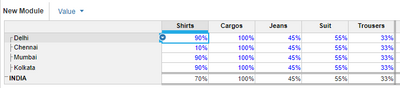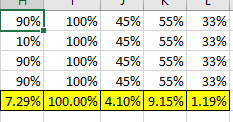2. Module 2... Line Item (COUNT) as 1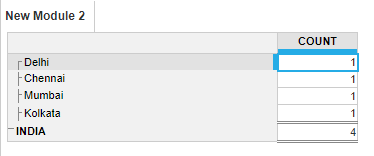3. Final Module  with Result.. All tallied except Shirts....(compare with the excel shown in 1st point)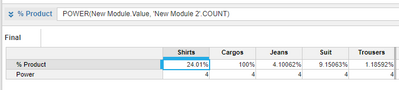2nd scenario:

Once I have changed the figure to 90% against Chennai in Input cell.. means Chennai + Shirts cell ...... all tallied..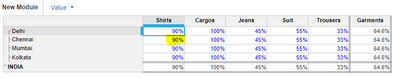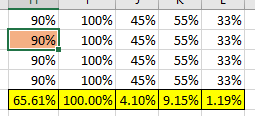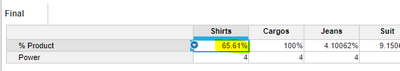Strange na!!

@DaanishSoomar  I hope have kept all modules and settings intact as per 3rd solution.. Share your thoughts

•Ok, I have a solution for you, check this out and see if this gets you home...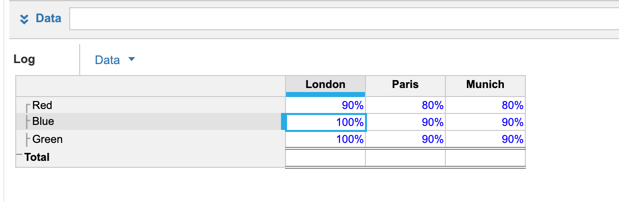Create two line items:

LN: LN(Data)

Final: ROUND(EXP(LN), 2, NEAREST, EXACT)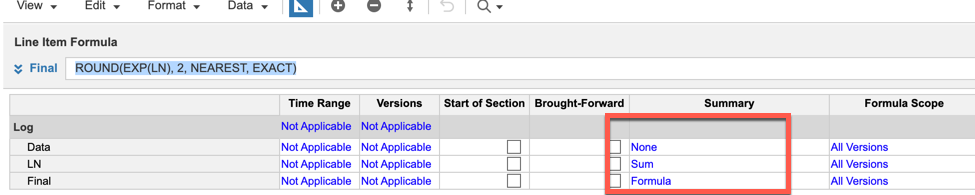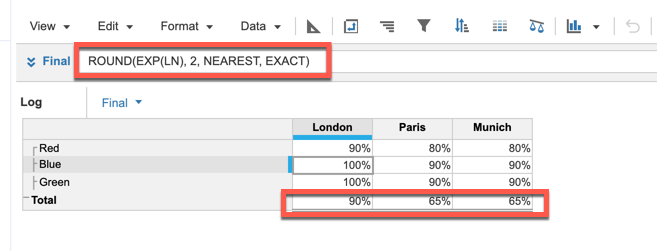See if that works for you.

Rob

•Hey @rob_marshall,

Very cool solution! I would be curious to see your thoughts on the difference from a PLANS standpoint in utilziing the POWER function from my post earlier as compared to the use of ROUND/LN functions in your example here.

Is 1 better than the other from a performance, processing, and sustainability standpoint?

•From a PLANS standpoint, not really.  The only real difference is I am doing all of the calcs within the same module (two of them) and not having to use a system module.  Both methods appear to work, so I would go with what is the most comfortable/logical to your way of thinking.

Rob

•Got it! Appreciate your insight @rob_marshall! I like your alternative approach as well 🙂